# Font Styles Paragraph Definition 1: Given La linear transformation from a vector space V into itself,...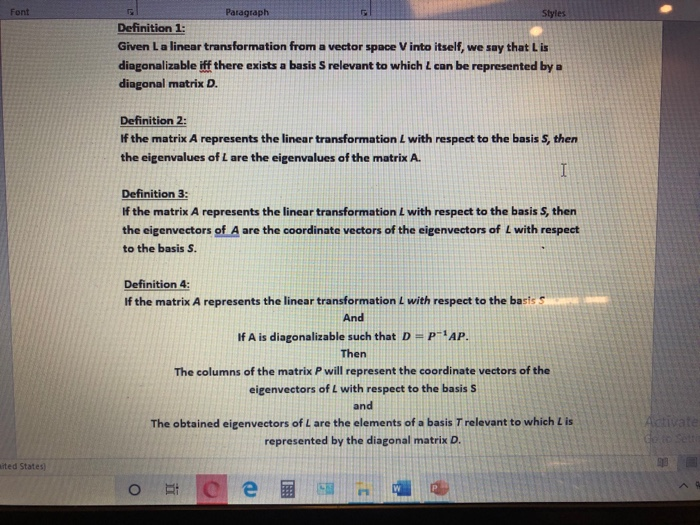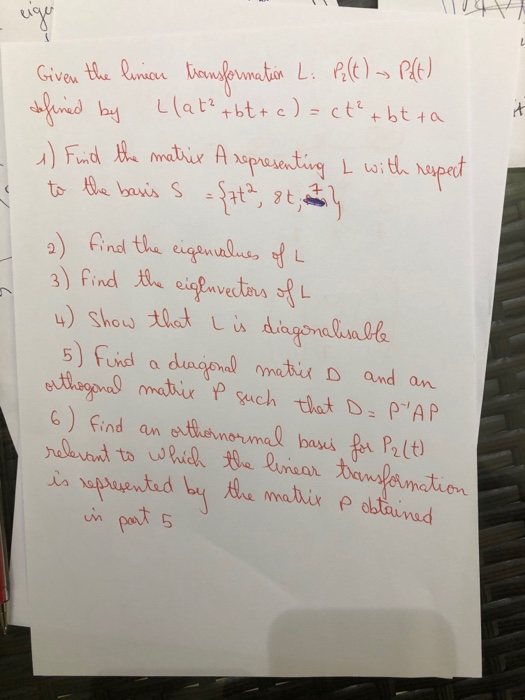Font Styles Paragraph Definition 1: Given La linear transformation from a vector space V into itself, we say that is diagonalizable iff there exists a basis S relevant to which can be represented by a diagonal matrix D. Definition 2: If the matrix A represents the linear transformation L with respect to the basis S, then the eigenvalues of L are the eigenvalues of the matrix A. I Definition 3: If the matrix A represents the linear transformation L with respect to the basis S, then the eigenvectors of A are the coordinate vectors of the eigenvectors of L with respect to the basis S. Definition 4 If the matrix A represents the linear transformation L with respect to the basis 5 And If A is diagonalizable such that D=p-1AP. Then The columns of the matrix P will represent the coordinate vectors of the eigenvectors of L with respect to the basis S and The obtained eigenvectors of L are the elements of a basis T relevant to which Lis represented by the diagonal matrix D. Activate ited States ORA e W
it way Given the linian transformation Li Pelt) - Palt) defined by Llat² tbt + c)=ct² tbt ta 1) Find the matrix A representing L with respect to the basis s ={pt2, 8th 2) find the eigenvalues of L 3) find the eigenvectors oft 4) show that I is diagonalisable 5) Find a diagonal matrix D and an orthogonal matrix P such that D=P"AP 6) find an orthernormal bases for P₂ (t) relevant to which the linear transformation! is represented by the matrix pobtained in part 5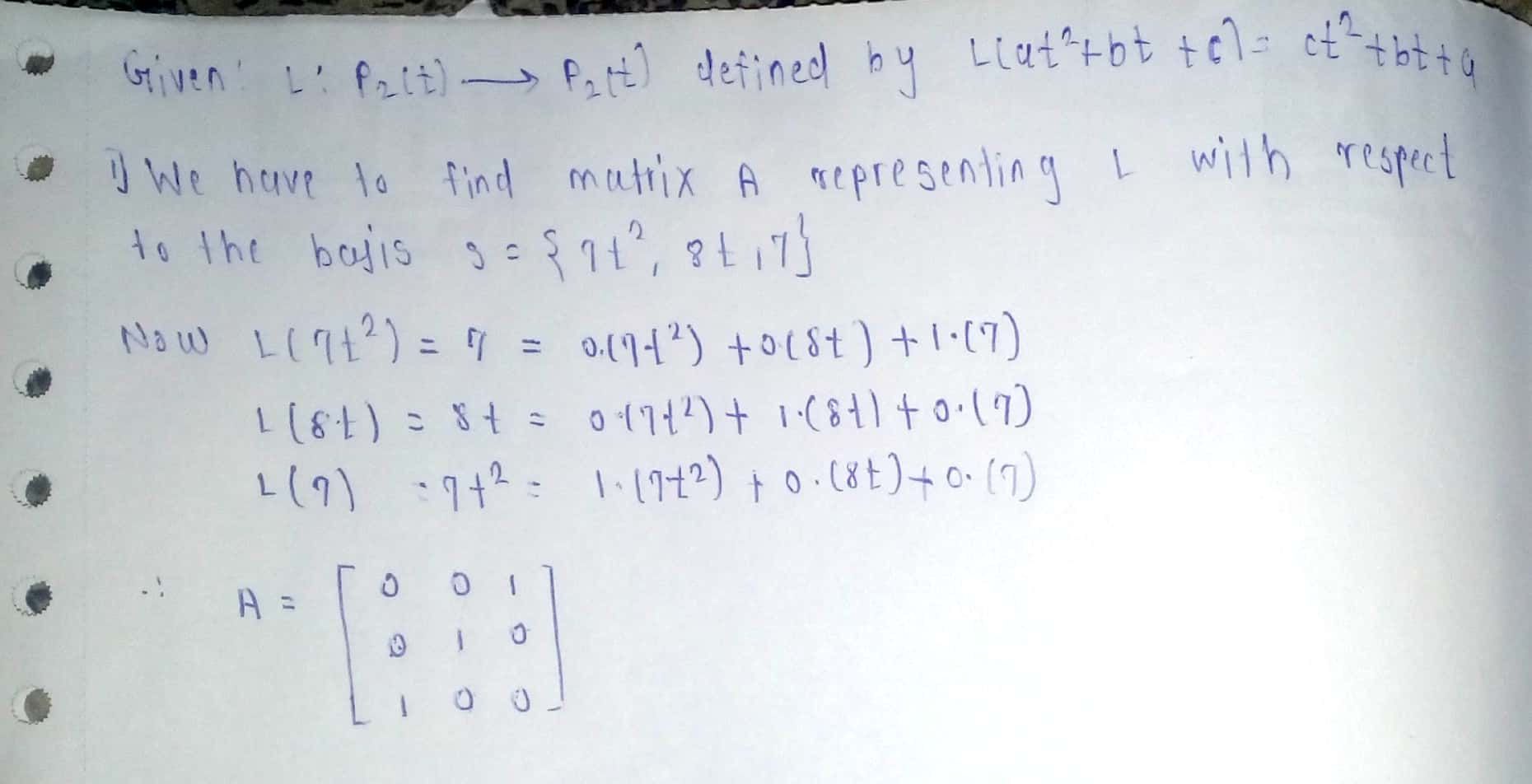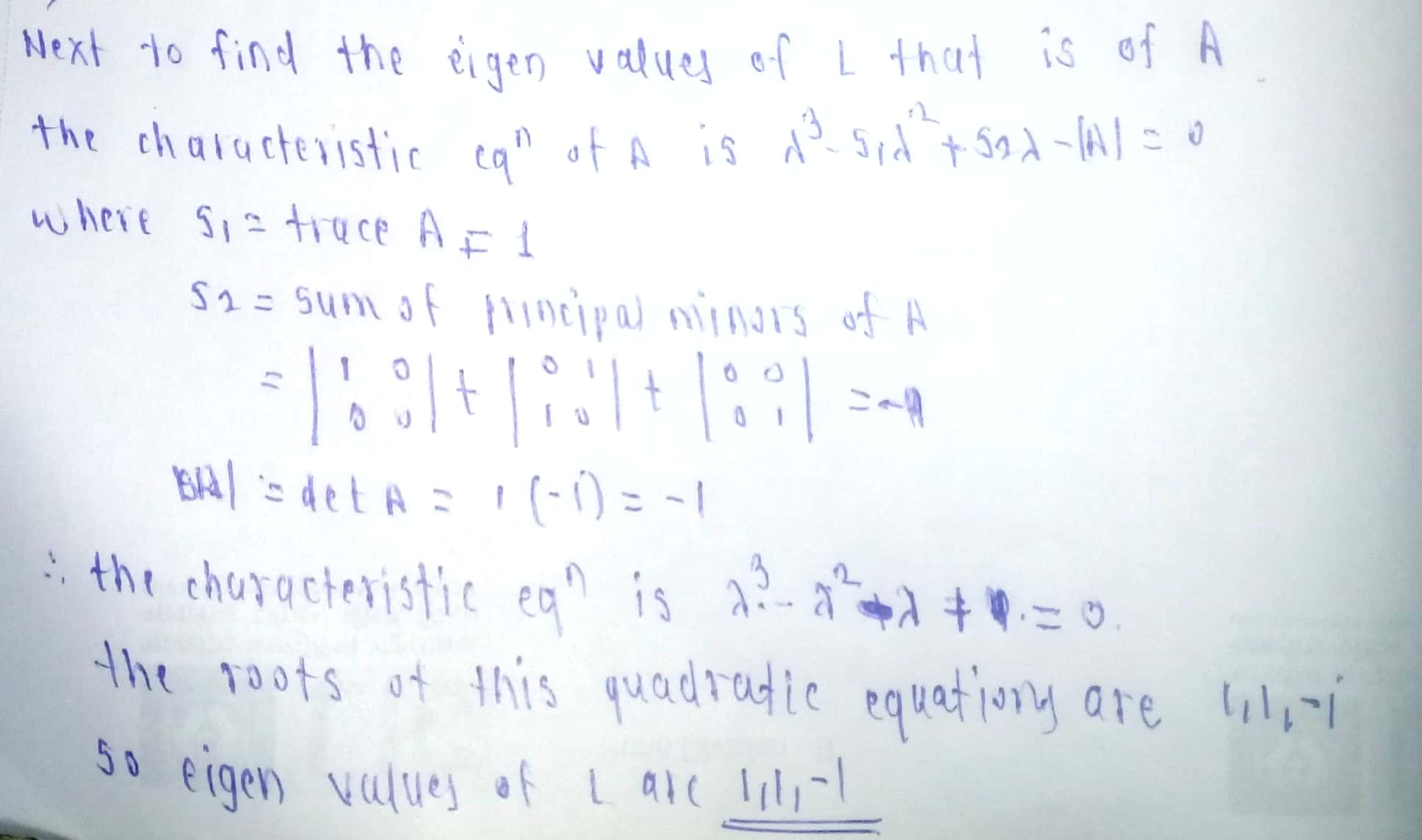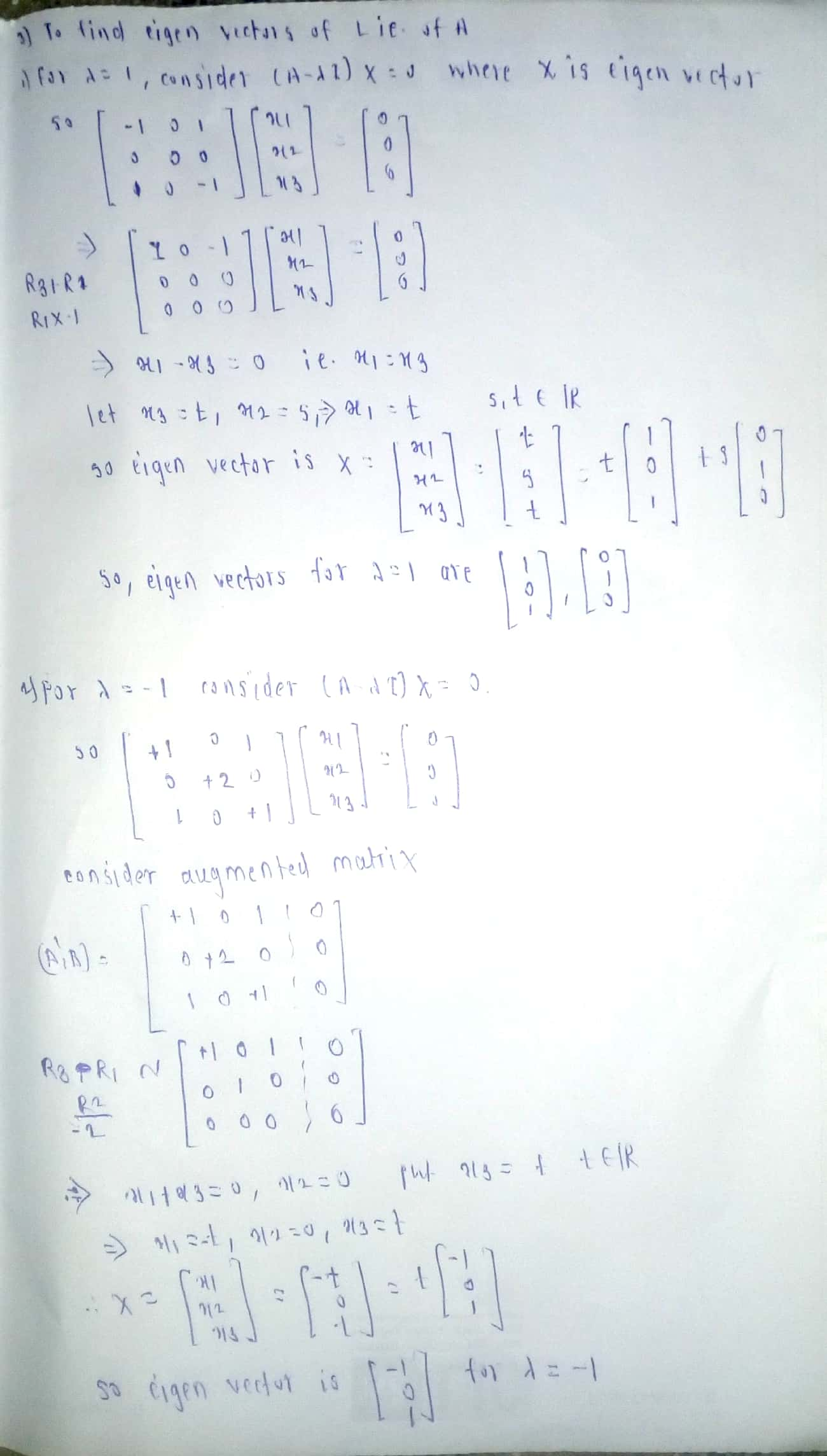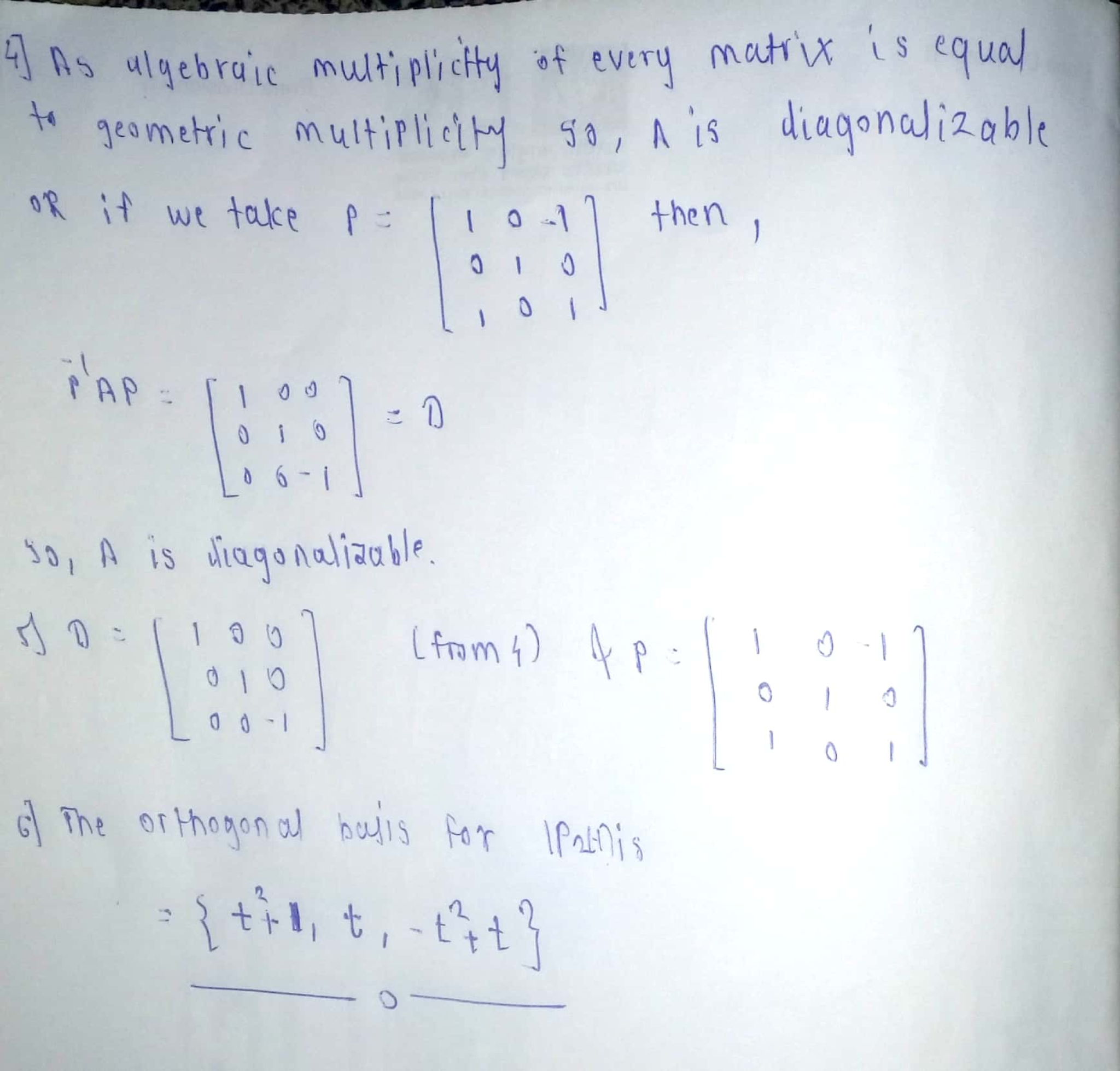#### Earn Coin

Coins can be redeemed for fabulous gifts.

Similar Homework Help Questions
• ### 1. Let L: P1(R) + P1(R) be a linear transformation given by L(a + bx) =...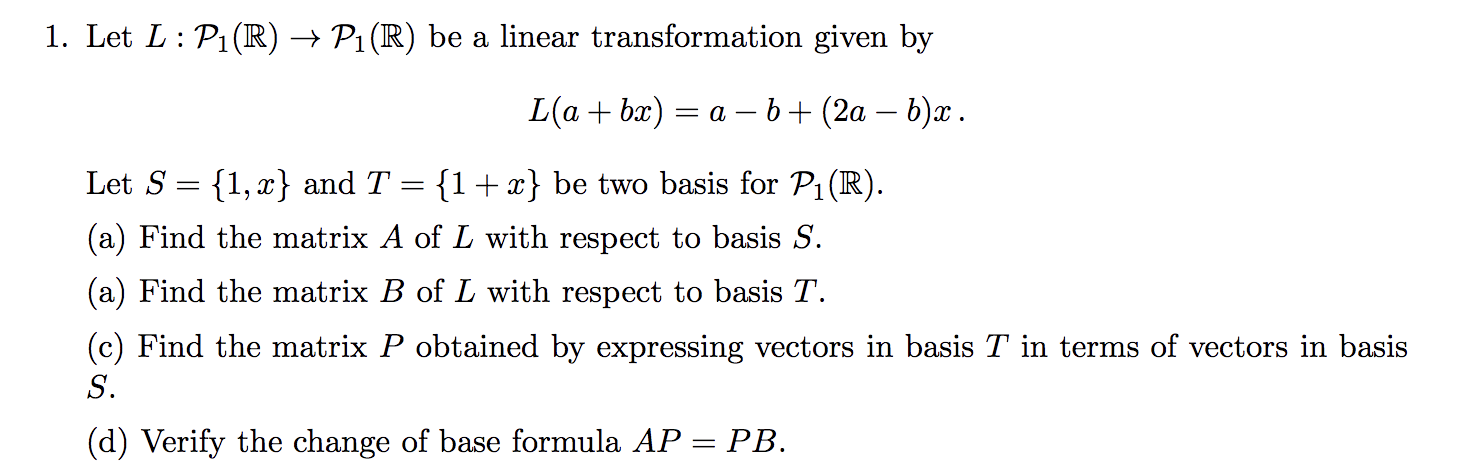1. Let L: P1(R) + P1(R) be a linear transformation given by L(a + bx) = a - b + (2a – b)x. Let S = {1, 2} and T = {1+x} be two basis for P1(R). (a) Find the matrix A of L with respect to basis S. (a) Find the matrix B of L with respect to basis T. (c) Find the matrix P obtained by expressing vectors in basis T in terms of vectors in basis (d)...

• ### 1 point) Read 'Diagonalization Changing to a Basis of Eigenvectors' before attempting this problem. Suppose that V is a 5-dimensional vector space. Let S -(vi,... , vs) be some ordered basis...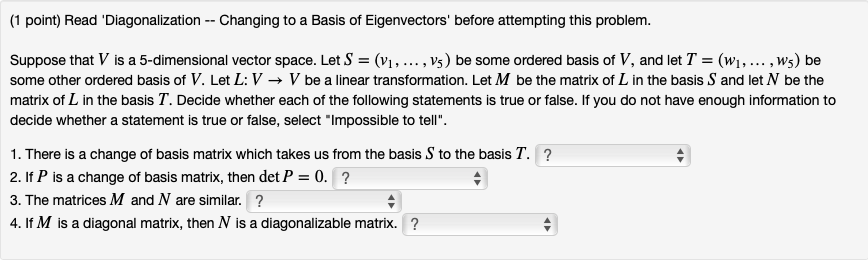1 point) Read 'Diagonalization Changing to a Basis of Eigenvectors' before attempting this problem. Suppose that V is a 5-dimensional vector space. Let S -(vi,... , vs) be some ordered basis of V, and let T-(wi.... . ws) be some other ordered basis of V. Let L: V → V be a linear transformation. Let M be the matrix of L in the basis Sand et N be the matrix of L in the basis T. Decide whether each of...

• ### (2) The matrix A-[-5 12 12 5 represents a reflection combined with a scaling by a factor of 13. (a) Find vectors ui and...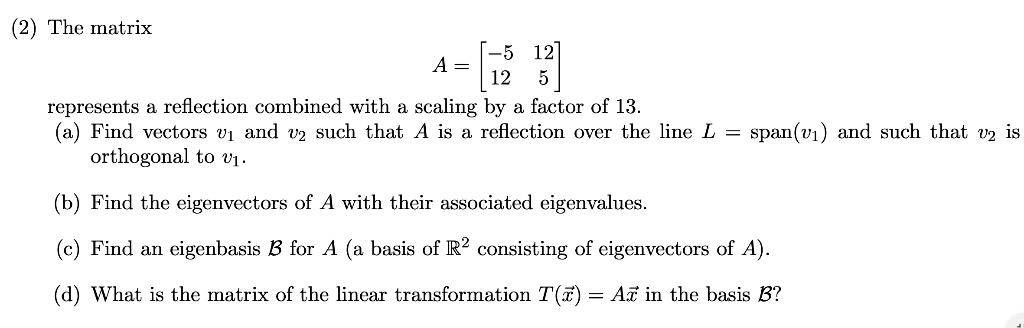(2) The matrix A-[-5 12 12 5 represents a reflection combined with a scaling by a factor of 13. (a) Find vectors ui and v2 such that A is reflection over the line L = span(n) and such that v2 is orthogonal to vi (b) Find the eigenvectors of A with their associated eigenvalues. (c) Find an eigenbasis B for A (a basis of R2 consisting of eigenvectors of A). (d) what is the matrix of the linear transformation T(z)...

• ### Please give a detailed explanation. I really need help understanding this. Thank you. (eigenvalues, eigenvectors) Let TA :R3-R3 be a linear transformation where 「1-4 TA(X)41-X. (1) Please find an ord...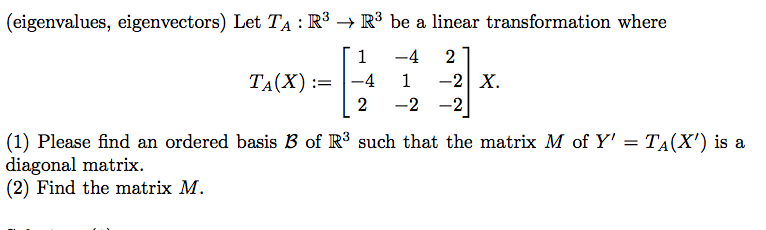Please give a detailed explanation. I really need help understanding this. Thank you. (eigenvalues, eigenvectors) Let TA :R3-R3 be a linear transformation where 「1-4 TA(X)41-X. (1) Please find an ordered basis B of R3 such that the matrix M of Y' - TA(X') is a diagonal matrix. (2) Find the matrix M. (eigenvalues, eigenvectors) Let TA :R3-R3 be a linear transformation where 「1-4 TA(X)41-X. (1) Please find an ordered basis B of R3 such that the matrix M of Y'...

• ### vectors pure and applied. exercise 6.4.2 OIK IIC rather than Example 6.4.1 Let ul, u2 be a basis for F2. The linear map β : F., p given by is non-diagonalisable. hat β is diagonali able with resp...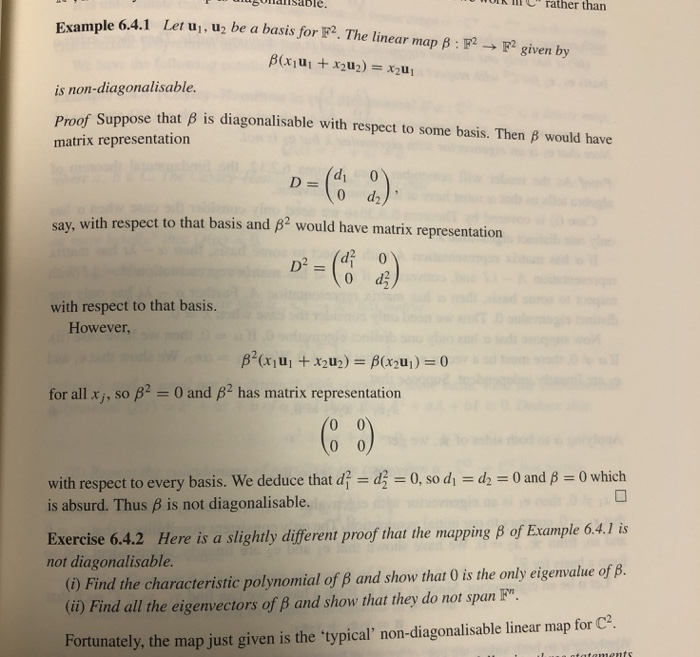vectors pure and applied. exercise 6.4.2 OIK IIC rather than Example 6.4.1 Let ul, u2 be a basis for F2. The linear map β : F., p given by is non-diagonalisable. hat β is diagonali able with respect to some basis. Then β would have Proof Suppose t matrix representation D=(d, 0 say, with respect to that basis and ß2 would have matrix representation 2 (d2 0 with respect to that basis. However for all xj, so β-0 and β2...

• ### 3. This example hopes to illustrate why the vector spaces the linear transformation are defined o...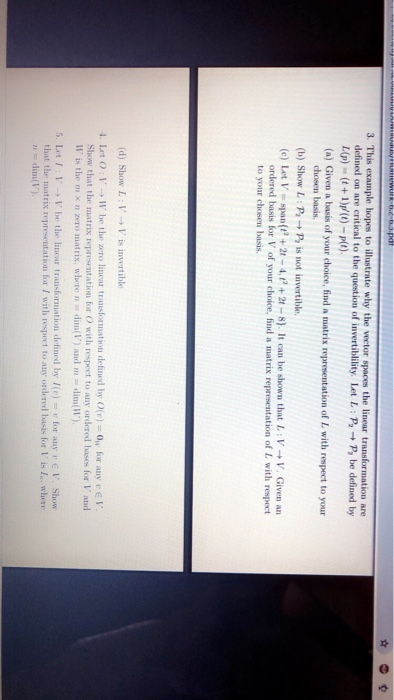3. This example hopes to illustrate why the vector spaces the linear transformation are defined on are critical to the question of invertibility. Let L : → p, be defined by L(p)(t+1)p(t)-plt). (a) Given a basis of your choice, find a matrix representation of I with respect to your chosen basis (b) Show L: P+P is not invertible (e) Let V-span+21-4,+2t-8). It can be shown that L VV. Given an ordered basis for V of your choice, find a matrix...

• ### How was the linear transformation of b1 and b2 were applied (L(b1) , L(b2))? NOTE: b1=(1,1)^T , b2=(-1,1)^T Linear Transformations EXAMPLE 4 Let L be a linear transformation mapping R? into itself an...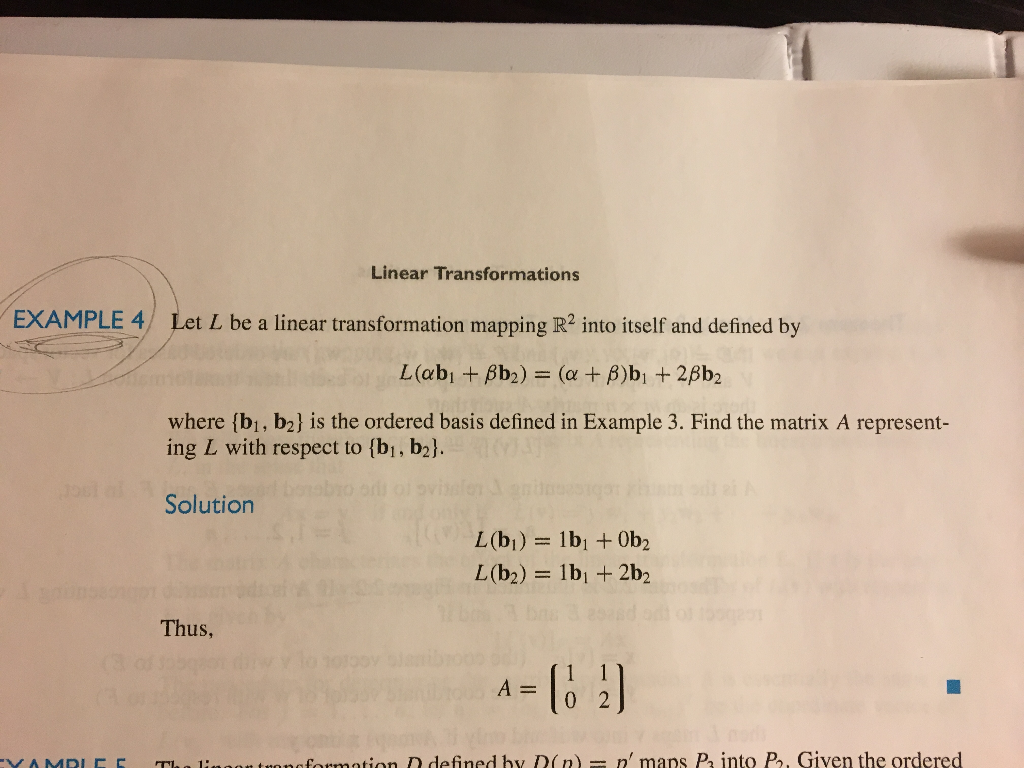How was the linear transformation of b1 and b2 were applied (L(b1) , L(b2))? NOTE: b1=(1,1)^T , b2=(-1,1)^T Linear Transformations EXAMPLE 4 Let L be a linear transformation mapping R? into itself and defined by where (bi, b2] is the ordered basis defined in Example 3. Find the matrix A represent- ing L with respect to [bi, b2l Solution Thus, A0 2 onofosmation D defined by D(n n' maps P into P, Given the ordered Linear Transformations EXAMPLE 4 Let...

• ### Let T be a linear operator on a finite dimensional vector space with a matrix representation...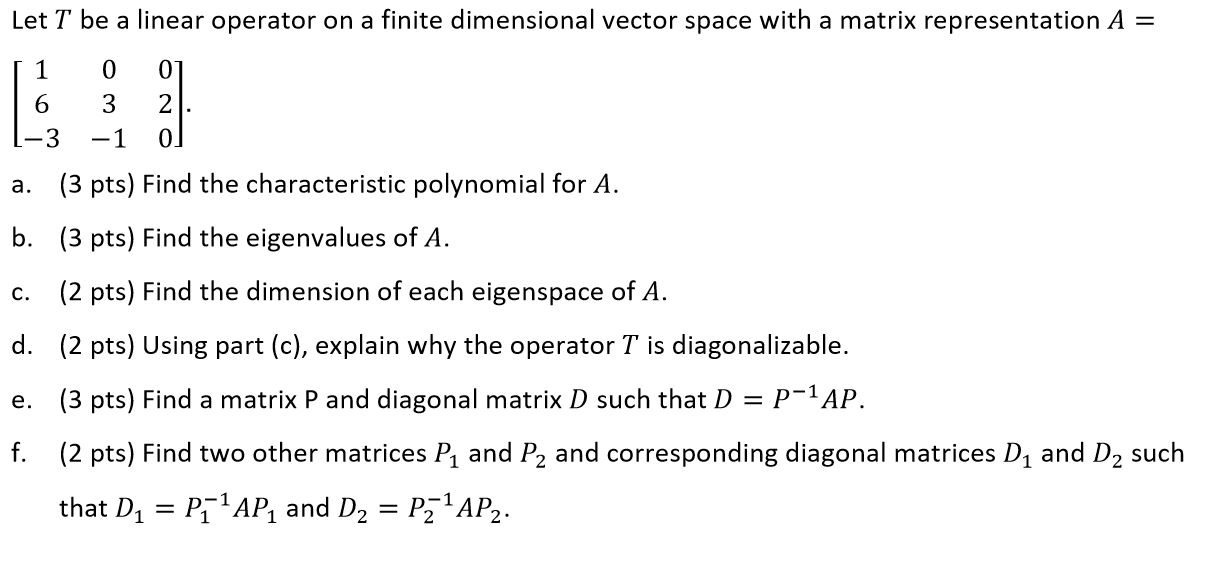Let T be a linear operator on a finite dimensional vector space with a matrix representation A = 1 1 0 0] 16 3 2 1-3 -1 0 a. (3 pts) Find the characteristic polynomial for A. b. (3 pts) Find the eigenvalues of A. C. (2 pts) Find the dimension of each eigenspace of A. d. (2 pts) Using part (c), explain why the operator T is diagonalizable. e. (3 pts) Find a matrix P and diagonal matrix D...

• ### 3. Consider the vector space V = R2[x] with its standard ordered basisE = 1,x,x2 and the linear map T :R2[x]−→R2[x], T(p...

3. Consider the vector space V = R2[x] with its standard ordered basisE = 1,x,x2 and the linear map T :R2[x]−→R2[x], T(p)=p(x−1)−p(0)x2 (a) (1 point) What is [T]E? (b) (1 point) Is T invertible? (c) (6 points) Compute the eigenvalues of T and their algebraic multiplicity. (d) (2 points) Is T diagonalisable? If so, find a matrix Q such that Q−1[T]EQ is diagonal. If not, findQ, so that the above matrix is upper triangular.

• ### -00)0) 2 (AB 22) Let L : R, R2 be a linear transformation. You are given...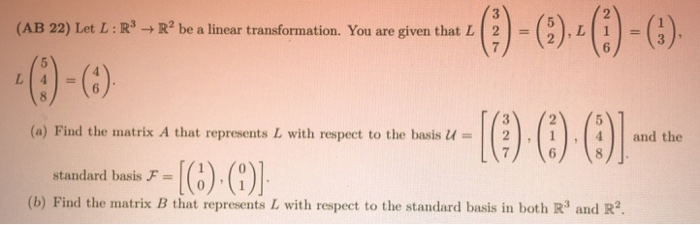-00)0) 2 (AB 22) Let L : R, R2 be a linear transformation. You are given that L 2- 3 (a) Find the matrix A that represents L with respect to the basisu-| | 2-1 1-1 4 1 and the 6 standard basis F1 (b) Find the matrix B that represents IL with respect to the standard basis in both R3 and R2